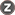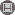Volume 17, Issue 1 (5-2018)                   TB 2018, 17(1): 14-23 | Back to browse issues page

BibTeX | RIS | EndNote | Medlars | ProCite | Reference Manager | RefWorks
Send citation to:askarishahi M, ghasemi N, Fallahzade H, Afkhami Ardekani M, AfkhamiArdekani A. Comparing of Decision Tree with Logistic Regression Model in Evaluating Osteoporosis. TB. 2018; 17 (1) :14-23
URL: http://tbj.ssu.ac.ir/article-1-1209-en.html
University , moasbio@gmail.com
Abstract:   (937 Views)
Introduction: Early detection of osteoporosis is a key to preventing of it; but recognition, without the use of appropriate diagnostic methods, due to the complexity of risk factors and gradual bone loss process, is problem. The purpose of this study is to develop and efficiency evaluation a predictive model of osteoporosis using decision tree technique as a diagnostic method based on available risk factors; thereby to identify individuals at risk for preventive activities.
Methods: In this study used data from 131 women aged 20 – 40 years. Response variable was amount of BMD (t-score) L1-L4 lumbar region that divided on two group, normal (t-score>= -1) and at risk of osteoporosis (t-score< -1). To determine risk factors of osteoporosis used from decision tree model with method of k- fold cross validation k=4 and logistic regression .To assess the accuracy prediction of two model, the area under receiver operative characteristic curves (AUROC) was used. Data analysis was performed by R software.
Results: Three variables number of pregnancies, BMI and calcium levels as risk factors for osteoporosis were obtained from the decision tree model and Area under receiver operative characteristic decision tree and logistic regression, respectively 0.665 and 0.686 were obtained.
Conclusion: Area under receiver operative characteristic curve showed advantage superiority of logistic regression that according to advantages of the decision tree applying simultaneously of two models is recommended.